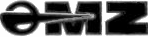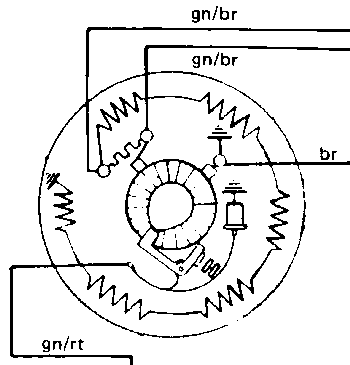# 6v - 12v Conversions

Home ] MZ Links ] MZ Main Index ] Search Site ] Join The MZ Riders ]

#### The good news, the conversion is simple. .

But there is a downside.

First, the conversion.

Replace all the bulbs with 12v items,
fit a 12v regulator, You could fit an electronic regulator . See the modifications for ETZ's, fairly straight forward.
a 12v coil,
a 12v horn,
a 12v indicator relay
and fit a 12v battery,
Check connections and polarity - start bike - it works, honestly. . . .

#### The downside. . .

###### The battery will not receive a charge unless the revs are above 3000 rpm.

A bit of a drawback for the naturally low revving MZ engine. So it cannot really be considered as a true 12v conversion.

Perhaps it would be helpful to look at the 6v versus 12v debate,

#### The Theory

It is generally accepted that a 12v system can offer advantages over a 6v one in terms of brighter lights, surer starting etc., but why is this the case?

We need to remind ourselves of a very important electrical relationship. It can be arranged in three ways, the chosen form being simply a matter of the most convenient application to the problem in hand.

W=V * A
V=W / A
A=W / V

V=Volts, A=Amps, W=Watts

We can now apply an appropriate form of this equation to two imaginary circuits, identical except for the fact one operates at 6v and the other at 12v. Virtually all electrical circuits can be thought of as a source of power, a load that consumes that power and the conductors which carry the power from the source to the load.

First, assume that the source of power is a 6v battery and the load is, say, a 60w light bulb designed to run from a 6v supply.
i.e. the bulb would be specified as a 6v 60w type. It can be calculated that the bulb will pass a current of 60w / 6v=10A . This current has to be carried by the conductors i.e. the wiring.

Our second circuit is identical except that it runs from a 12v battery and therefore uses a 12v 60w bulb. This time the current carried by the wiring will be 60w / 12v=5A . It can be seen for a given wattage load the current flowing in 12v system will be half the that of its 6v equivalent.

It can also be mentioned here that other things being equal, the brightness of the bulb depends entirely on its wattage. Regardless of whether this is derived from the low voltage/high current or high voltage/low current circuit. Going on the extent of the principle discussed so far, there should be no difference between our two hypothetical systems. However this is not the whole story.

To see where the differences lie we need to look at another fundamental electrical relationship. No electrical conductor is perfect (except for some very odd things that happen at temperatures around absolute zero. Despite your winter biking experience, it never gets that cold, and can be ignored by us).

All conductors oppose the flow of an electrical current passing through them. They are said to possess resistance. The nature of electrical resistance was investigated by the German physicist, Georg Simon Ohm, who in 1827 put forward the law that now carries his name.

Ohms law states, in essence, that for a given conductor, it takes a certain voltage to send a particular current through it. If it takes one volt to make a current of one amp flow the conductor is said to possess a resistance of one ohm (represented by the Greek omega symbol).
Ohms law can be written as,

R=V / A
V=A * R
A=V / R

R=Resistance

The resistance of the wiring and connectors etc. linking the headlamp of a motorcycle to the battery could easily be in the order of 0.1 ohm, particularly if the connectors or contacts are in poor condition. So lets go back to our original examples, but this time introducing a 0.1 ohm resistance into the circuits.

In the case of the 6v 60w system the bulb will be trying to take 10A which would cause a voltage loss that can be calculated with Ohms law, 10A * 0.1 ohm = 1v. The bulb is now only getting 5v instead of the correct 6v. Whilst, as mentioned earlier, it is the power that determines its brightness, the power itself is dependent on the bulb receiving its specified voltage. Further, whilst the mathematical treatment starts to get a bit complicated and will therefore be ignored here, it can be said that a reduction in voltage of the supply to a bulb will actually cause a loss of power greater in proportion than the loss of voltage. It is very likely that a loss of 1/6 th of its correct supply voltage will have a drastic effect upon the brightness of our 6v 60w bulb.

Now, in contrast, take the 12v system. This time the current, as we have seen, is 5A and again by applying Ohm's law, the voltage lost in 0.1 ohm wiring resistance will be 5A * 0.1 ohm = 0.5v. The bulb will therefore be receiving 11.5v. However, 0.5v is only 1/24 th of the correct 12v. Whilst the loss is not desirable it is only a quarter of the loss by the 6v system for the same amount of resistance. In fact a voltage loss of 1/24 th will probably have no noticeable effect on the brightness of the bulb.

It can be seen for a given power rating, a high voltage/low current system is much less affected by the inevitable wiring resistance than its low voltage/high current counterpart.

Another way of putting it is, if you have a 6v system it is much more important to keep the wiring, connectors and switches in the best possible condition. Such a system will work well if sufficient care is taken with its maintenance.

#### The Facts Behind a 6v to 12v Conversion

The first part of this account attempted to show why a 12v system is preferred and why a conversion, on the face of things, is a desirable move for someone who has an older model MZ. However we need to examine what these conversions involve.

Accepted wisdom has it that all you need to do to your 6v system is replace the battery, regulator, coil, horn and indicator relay with the equivalent 12v items. This is true but there is one important omission, the 6v dynamo. Again accepted wisdom comes to our rescue and says that the dynamo replacement is not necessary as the regulator and battery control the voltage supplied to the rest of the system. Once again this is true, but the fly in ointment is we now need to maintain a higher rpm to keep the battery charged. To see why this is the case it's necessary to consider the physical principle upon which the dynamo works.

The electromagnetic principles in question were discovered and investigated by Michael Faraday in the 1830's. What has now become known (surprisingly) as Faraday's Law states that when an electrical conductor passes through a magnetic field, a voltage is produced across the ends of the conductor, which is directly proportional to the strength of the field, multiplied by the rate at which this conductor cuts through the field, multiplied by the length of the conductor in the field.
Because of the direct proportionality altering any one of those factors by a given amount will affect the result, (the Voltage generated). Altering more than one will have a combined effect on the outcome.

Now apply these relationships to our practical dynamo.

1. The magnetic field is provided by the field coils and for a given current in the coils, the field will be constant.
2. The conductor cutting through the field is the armature (rotor) winding and the rate at which the conductor cuts the field is the rate of rotation of the armature i.e. engine rpm.
3. The length of the conductor is, in practice, the number of turns on the armature winding

For a given dynamo, let us assume that item 1. (the field) is kept constant. Item 3. (the armature windings) has to remain constant as it part of the dynamo construction, but item 2. (rpm) can be changed at will. It can thus be seen that with the current in the field coils kept constant the voltage produced is directly proportional to the speed of rotation.

In this sense it can be said that specifying the output voltage of a dynamo as 6v or 12v is meaningless, unless the speed of rotation is also stated. In fact the voltage will rise rather faster than the speed of rotation as the increasing voltage will tend to cause the current and thus the field strength to increase as well.

In practice a dynamo that provides electrical power for a motorcycle will be designed to provide its specified voltage at a little over idle speed.

In fact , a 6v dynamo will probably give about 7v and similarly a 12v dynamo about 14v. From what was said earlier, it can be seen that if the rpm is doubled, the output of a 7v 1500 rpm dynamo would become 14v at 3000 rpm and 28v at 6000 rpm and so on until the whole plot goes bang! Naturally this can not be allowed to happen as the bulbs, battery etc. would self destruct.It is the job of the regulator to control the dynamo output and maintain it at its correct 7v across the engine speed range. It does this by sensing the output of the dynamo and reducing the field coils current accordingly, which in turn reduces the strength of the magnetic field in which the armature rotates. Thus reducing the voltage created.

It is obvious from the above that it is indeed possible to convert 6v to 12v whilst retaining the same dynamo. What you are doing in effect is shifting the dynamo operating point from its normal 7v 1500 rpm mode to a 14v 3000 rpm mode. The downside is the minimum charging speed is now 3000 rpm or thereabouts.

To provide a true 12v conversion, the dynamo itself would have to be changed or modified.

The question is, can it be done?

Many thanks to John Higgon, Nigel Wright and especially John Luker.